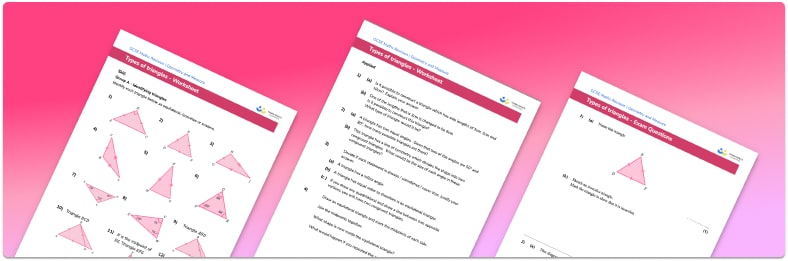# Types Of Triangles Worksheet• Section 1 of the types of triangles worksheet contains 20+ skills-based types of triangles questions, in 3 groups to support differentiation
• Section 2 of the classifying triangles worksheet contains 3 applied types of triangles questions with a mix of word problems and deeper problem solving questions
• Section 3 contains 3 foundation and higher level GCSE exam style types of triangles questions
• Answers and a mark scheme for all types of triangles questions are provided
• Questions follow variation theory with plenty of opportunities for students to work independently at their own level
• All questions created by fully qualified expert secondary maths teachers
• Suitable for GCSE maths revision for AQA, OCR and Edexcel exam boards

• This field is for validation purposes and should be left unchanged.

You can unsubscribe at any time (each email we send will contain an easy way to unsubscribe). To find out more about how we use your data, see our privacy policy.

### Types of triangles at a glance

There are four different types of triangles that we need to know about. Equilateral triangles have 3 equal side lengths and 3 equal angles, isosceles triangles have 2 equal sides and 2 equal angles whilst scalene triangles have sides and angles which are all different. Right angle triangles, sometimes called right triangles, are triangles which contain a right angle. Right angle triangles can be isosceles or scalene.

Triangles can contain acute angles, obtuse angles or right angles. Since the angles of a triangle add up to 180°, a triangle can contain at most one right angle or obtuse angle. A triangle with an obtuse angle is called an obtuse triangle and a triangle where all three angles are acute is called an acute triangle.

Looking forward, students can then progress to additional polygons worksheets and other geometry worksheets, for example a simplifying expressions worksheet or simultaneous equations worksheet.For more teaching and learning support on Geometry our GCSE maths lessons provide step by step support for all GCSE maths concepts.

## Do you have KS4 students who need more focused attention to succeed at GCSE?There will be students in your class who require individual attention to help them succeed in their maths GCSEs. In a class of 30, it’s not always easy to provide.

Help your students feel confident with exam-style questions and the strategies they’ll need to answer them correctly with our dedicated GCSE maths revision programme.

Lessons are selected to provide support where each student needs it most, and specially-trained GCSE maths tutors adapt the pitch and pace of each lesson. This ensures a personalised revision programme that raises grades and boosts confidence.

Find out more